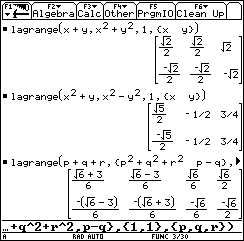## Texas Instruments TI-89 and TI-92 Plus Computer

Last page update: December 21, 1999
 Lagrange Optimization Function - for the TI-89 and TI-92 Plus Version 1.1   (December 16, 1999) Download [ lagrange.zip] Description Uses Lagrange Multipliers for optimization.  1.1: Modified function to allow easy language translation. see . Screen shotsDocumentation Usage: lagrange(ObjectiveExpr,ConstraintExpr,ConstraintVal,VarList)  ObjectiveExpr: The objective function. ConstraintExpr: The constraint expression or a list of constraint expressions. Does not need to be a list if there is only one constraint. ConstraintVal: The value of the constraint expression. This must be zero if the value has already been subtracted from the constraint expression. For example: lagrange(x+y, x2+y2, 1, {x,y}) or lagrange(x+y, x2+y2-1, 0, {x,y}). Does not need to be a list if there is only one constraint. VarList: The list of variables in the functions.  Return value: A matrix where each row of the matrix is a solution. The last column of the matrix is ObjectiveExpr evaluated at the point specified by the preceding columns.  Examples: 1. Find the maximum and minimum values of x+y subject to the constraint x2+y2=1:    lagrange( x+y, x2+y2, 1, {x,y})     [[sqrt(2)/2,   sqrt(2)/2,  sqrt(2)]      [-sqrt(2)/2, -sqrt(2)/2, -sqrt(2)]]   Row 1: x =  sqrt(2)/2,   y =  sqrt(2)/2,  x+y =  sqrt(2)  Maximum   Row 2: x = -sqrt(2)/2,   y = -sqrt(2)/2,  x+y = -sqrt(2)  Minimum